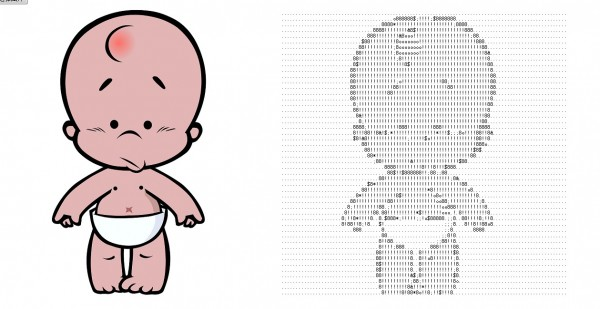# 通过javascript把图片转化为字符画发布者 ourjs  发布时间 1382577258000

#### 1.获取上传图片对象数据• Internet Explorer: 10+
• Firefox: 10+
• Chrome: 13+
• Opera: 12+
• Safari: partial

`var reader = new FileReader(); //建立一个FileReader接口 reader.readAsDataURL(fileBtn.files); //fileBtn为文件上传控件对象 reader.onload = function () { //在onload事件中访问图像数据  img.src = reader.result; }`

#### 2.获取图像对象像素点

`var imgData = c.getImageData(0, 0, img.width, img.height); var imgDataArr = imgData.data; var imgDataWidth = imgData.width; var imgDataHeight = imgData.height; for (h = 0; h < imgDataHeight; h += 12) {  for (w = 0; w < imgDataWidth; w += 6) {  var index = (w + imgDataWidth * h) * 4;  var r = imgDataArr[index + 0];  var g = imgDataArr[index + 1];  var b = imgDataArr[index + 2];  } } `

#### 3.根据rgb值计算灰度

##### 1、简化 sRGB IEC61966-2.1 [gamma=2.20]
`Gray = (R^2.2 * 0.2126  + G^2.2  * 0.7152  + B^2.2  * 0.0722)^(1/2.2)`
##### 2、 Adobe RGB (1998) [gamma=2.20]
`Gray = (R^2.2 * 0.2973  + G^2.2  * 0.6274  + B^2.2  * 0.0753)^(1/2.2)`
##### 3、Apple RGB [gamma=1.80]
`Gray = (R^1.8 * 0.2446  + G^1.8  * 0.6720  + B^1.8  * 0.0833)^(1/1.8)`
##### 4、ColorMatch RGB [gamma=1.8]
`Gray = (R^1.8 * 0.2750  + G^1.8  * 0.6581  + B^1.8  * 0.0670)^(1/1.8)`
##### 5、简化 KODAK DC Series Digital Camera [gamma=2.2]
`Gray = (R^2.2 * 0.2229  + G^2.2  * 0.7175  + B^2.2  * 0.0595)^(1/2.2)`

// 根据rgb值计算灰度
`// 根据rgb值计算灰度 function getGray(r, g, b) {  return 0.299 * r + 0.578 * g + 0.114 * b; } `

#### 4.根据灰度生成相应字符

`// 根据灰度生成相应字符 function toText(g) {  if (g <= 30) {  return ’8′;  } else if (g > 30 && g <= 60) {  return ’&’;  } else if (g > 60 && g <= 120) {  return ’\$';  } else if (g > 120 && g <= 150) {  return ’*';  } else if (g > 150 && g <= 180) {  return ’o';  } else if (g > 180 && g <= 210) {  return ’!';  } else if (g > 210 && g <= 240) {  return ’;';  } else {  return ‘.’;  } } `

##### 开源的 OurJS
OurJS开源博客已经迁移到 OnceOA 平台。

##### 关注我们

OnceJS